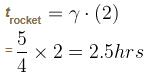Courses

# Special Theory Of Realativity MSQ Level – 2

## 10 Questions MCQ Test Topic wise Tests for IIT JAM Physics | Special Theory Of Realativity MSQ Level – 2

Description
This mock test of Special Theory Of Realativity MSQ Level – 2 for IIT JAM helps you for every IIT JAM entrance exam. This contains 10 Multiple Choice Questions for IIT JAM Special Theory Of Realativity MSQ Level – 2 (mcq) to study with solutions a complete question bank. The solved questions answers in this Special Theory Of Realativity MSQ Level – 2 quiz give you a good mix of easy questions and tough questions. IIT JAM students definitely take this Special Theory Of Realativity MSQ Level – 2 exercise for a better result in the exam. You can find other Special Theory Of Realativity MSQ Level – 2 extra questions, long questions & short questions for IIT JAM on EduRev as well by searching above.
*Multiple options can be correct
QUESTION: 1

### Consider two observers. One on the ground and another on a moving train. Both of them have clocks. Choose the correct statements.

Solution:

From the rest frame of the ground observer the clock in the train is moving. The train clock runs slow from the point of view of the ground observer. From the rest frame of the train observer, the ground clock appears to be moving
∴ From the reference frame of the train, the ground clock runs slow

The correct answers are: The ground observer finds the train clock run slow., The train observer finds the ground clock run slow.

*Multiple options can be correct
QUESTION: 2

### Which of the following statements is true?

Solution:

Explanation : b) mass of a photon is due to its momentum. When photon comes to rest velocity becomes zero thus mv=p=0. Hence rest mass of a photon is zero.

c) Rest mass (m0) is defined as the mass of the object at rest (or in rest frame) which remains constant.

Only the mass of the moving object changes as the speed increases  via m = m0/(1 - v2/c2)1/2

*Multiple options can be correct
QUESTION: 3

### Choose the correct statement

Solution:

It is possible that neither obeys special theory of relativity but they can obey under some conditions.

*Multiple options can be correct
QUESTION: 4

Which of the following are lorentz transformations?

Solution:

In physics, the Lorentz transformations are a one-parameter family of linear transformations from a coordinate frame in spacetime to another frame that moves at a constant velocity (the parameter) relative to the former. The respective inverse transformation is then parametrized by the negative of this velocity.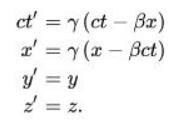In the transformation for x  and  t,  the coefficient of x  and  t,  is the same!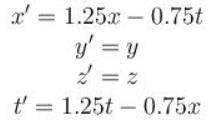*Multiple options can be correct
QUESTION: 5

In electrodynamics, the set of transformation rules for components of  E  and B  are given as follows :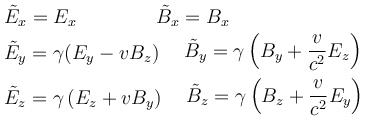Here ' ~' denotes the components of E and B in a reference frame moving along the x-axis with speed v

Choose the correct statement.

Solution:

Consider the dot product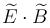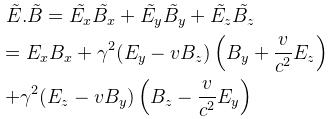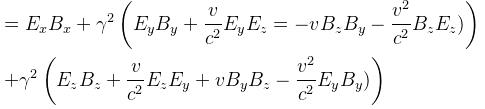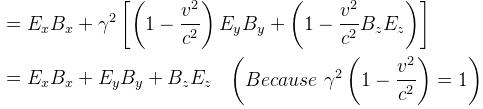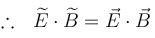∴  E.B is relativistically in invariant

Consider the quantity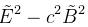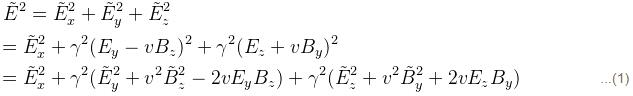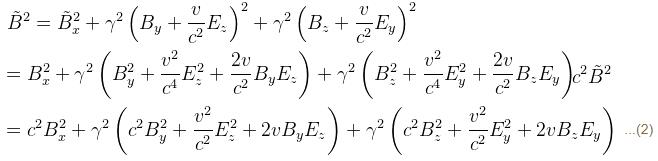Now (1) – (2)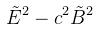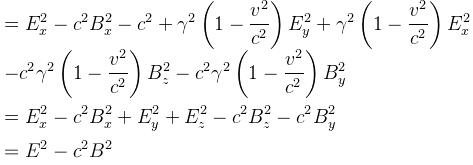Invariant !
The correct answers are: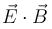is a relativistically invariant quantity, E2 - B2cis a relativistically invariant quantity

*Multiple options can be correct
QUESTION: 6

Tau leptons are observed to have an average half life of  Δt1 in the frame S1 in which the leptons are at rest. In an inertial frame S2 which is moving at a speed V12 relative to S1, the leptons are observed to have an average half life of  Δt2. In another inertial reference frame S3, which is moving at a speed V13 relative to S1  and at a speed V23 relative to S2, the leptons have an observed half life of Δt3. Which of the following is true?

Solution:

S1 is at rest
∴  lifetime is least in this frame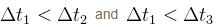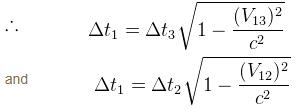The correct answers are: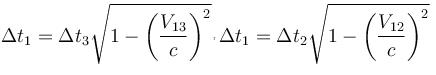*Multiple options can be correct
QUESTION: 7

According to Einsteins postulates of special theory of relativity

Solution:

The two postulates are:
The laws of physics are the same and can be stated in their simplest form in all inertial frames of reference.
The speed of light c is a constant, independent of the relative motion of the source.

To clarify the doubt:
The speed of light is a constant c = 3.00 × 108 m/s in a vacuum. If you remember the effect of the index of refraction from The Law of Refraction, the speed of light is lower in matter.

*Multiple options can be correct
QUESTION: 8

According to Einstein’s Special Theory of Relativity, laws of physics can be formulated based on ____________

Solution:

One of the postulates of Einstein’s Special Theory of Relativity states that all the inertial frames are equivalent to the formulation of laws of physics. Thus, it is suitable for all the inertial frames.

QUESTION: 9

Choose the correct statement

Solution:

Particles traveling at speeds close to the speed of light disintegrate slower than when they are at rest” is more apt when compared to “The life time of particles is greater when they are moving at speeds comparable to c

*Multiple options can be correct
QUESTION: 10

A rocket ship leaves earth at a speed of 3/5 c.When a clock on the rocket says 1 hour has elapsed, the rocket ship sends a light signal back to earth. Choose the correct statement (s)

Solution:

Rocket runs slow so earth clock reads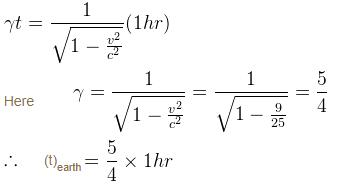∴  Earth clock read 1 hr 15 minutes.

By the earth observer, the rocket is now at a distance of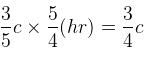hours away.
∴